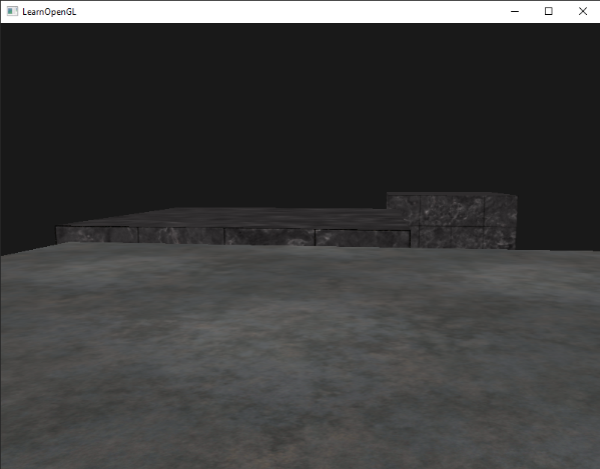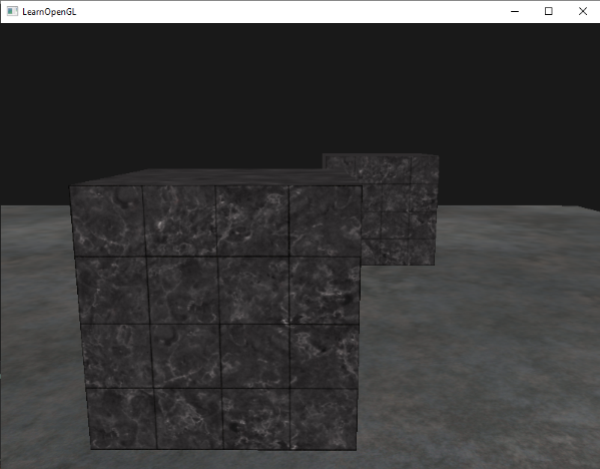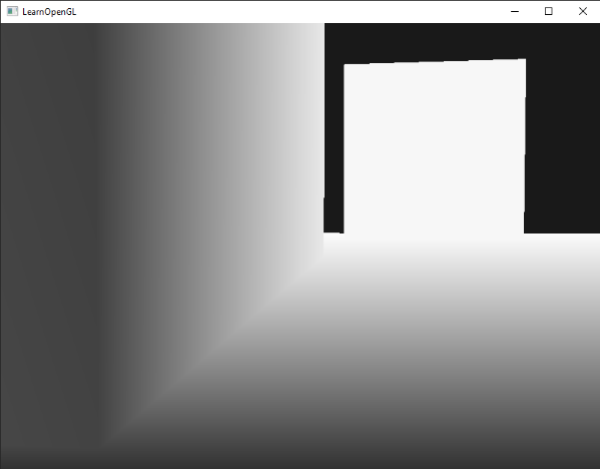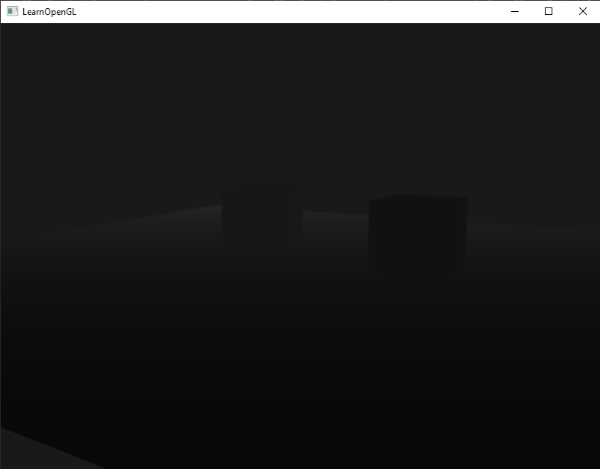# 深度测试

Important

glEnable(GL_DEPTH_TEST);


glClear(GL_COLOR_BUFFER_BIT | GL_DEPTH_BUFFER_BIT);


glDepthMask(GL_FALSE);


## 深度测试函数

OpenGL 允许我们修改它深度测试使用的比较运算符(comparison operators)。这样我们能够控制OpenGL通过或丢弃碎片和如何更新深度缓冲区。我们可以通过调用glDepthFunc来设置比较运算符 (或叫做深度函数(depth function)):

glDepthFunc(GL_LESS);


GL_ALWAYS 永远通过测试
GL_NEVER 永远不通过测试
GL_LESS 在片段深度值小于缓冲区的深度时通过测试
GL_EQUAL 在片段深度值等于缓冲区的深度时通过测试
GL_LEQUAL 在片段深度值小于等于缓冲区的深度时通过测试
GL_GREATER 在片段深度值大于缓冲区的深度时通过测试
GL_NOTEQUAL 在片段深度值不等于缓冲区的深度时通过测试
GL_GEQUAL 在片段深度值大于等于缓冲区的深度时通过测试

glDepthFunc(GL_ALWAYS);## 深度值精度Important## 深度缓冲区的可视化

void main()
{
color = vec4(vec3(gl_FragCoord.z), 1.0f);
}float z = depth * 2.0 - 1.0;


float linearDepth = (2.0 * near) / (far + near - z * (far - near));


#version 330 core

out vec4 color;

float LinearizeDepth(float depth)
{
float near = 0.1;
float far = 100.0;
float z = depth * 2.0 - 1.0; // Back to NDC
return (2.0 * near) / (far + near - z * (far - near));
}

void main()
{
float depth = LinearizeDepth(gl_FragCoord.z);
color = vec4(vec3(depth), 1.0f);
}## 深度冲突